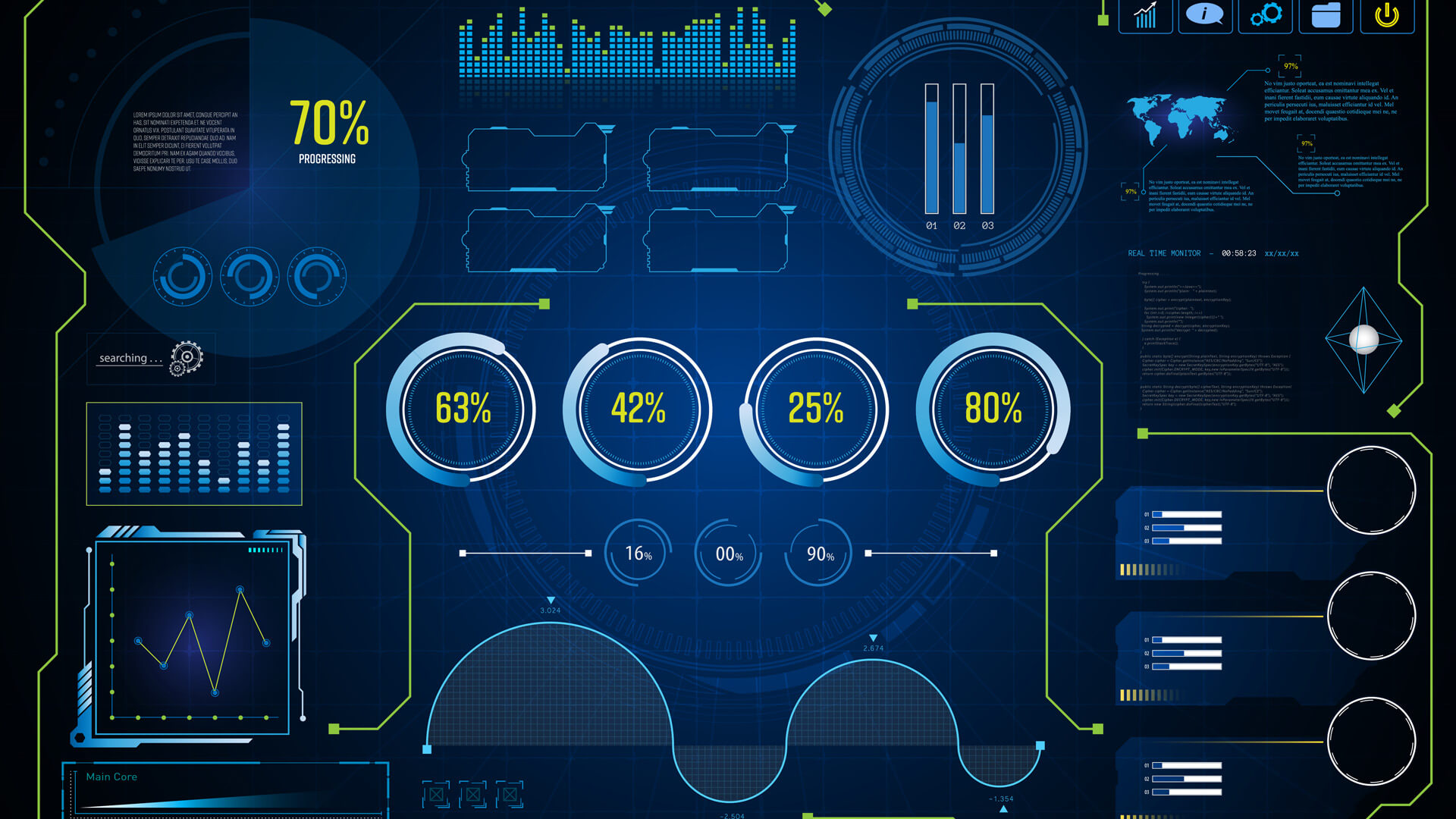## Control Systems (ECE) by Mr. B. Raja Gopal Reddy M.E, (Ph. D) This course covers concepts on block diagrams, time domain analysis solutions to time invariant Systems. It covers, in-depth, different aspects of stability analysis in frequency domain and time domain. Code : CSYJHECE31 Recommended For : JNTU B. Tech ECE 3rd Year 1st Semester

#### After Learning this subject,you should be able to

• After going through this course the student gets a through knowledge on open loop and closed loop control systems, concept of feedback in control systems, mathematical modeling and transfer function derivations of Synchros, AC and DC servo motors, Transfer function representation through block diagram algebra and signal flow graphs, time response analysis of different ordered systems through their characteristic equation and time domain specifications, stability analysis of control systems in S-domain through R-H criteria and root-locus techniques, frequency response analysis through bode diagrams, Nyquist, polar plots and the basics of state space analysis, design of PID controllers, lag, lead, lag-leadcompensators, with which he/she can able to apply the above conceptual things to real-world electrical and electronics problems and applications.

## Curriculum 21:10:11   units   |   9

• 1:35:46

UNIT 1.1
Control Systems: Introduction, Concepts of Control Systems Part 1, Concepts of Control Systems Part 2, Different examples of control systems, Breif History, Classification of control systems, Feed-Back Characteristics- part 1, Feed-Back Characteristics- Part 2, Open Loop and closed loop control systems and their differences, Mathematical models, laplace transforms, Advantages and disadvantages of transfer function, Differential equations

• 1:43:00

UNIT 1.2
Block digrams Representations: Block digrams Representations & rules- part 1, Block digrams rules- part 2, Block digrams of electrical systam, Block digrams Probelms - 1, Block digrams Probelms - 2, Block digrams Probelms - 3, Signal flow graph, Mason gain formula, Signal flow graph problems-2, Signal flow graph from block digram problems-3, Signal flow graph of RLC circuit problems-4, Signal flow graph problems-5, Signal flow graph from block digrams and differences

• 2:25:23

UNIT 2.1
Time Response Analysis: Introduction, test signals, Typs of TF,characteristic equation, Time response of 1st order system Inroduction, Impulse & step response of 1st order system, Ramp response of 1st order system, Time response of 2nd order system, Classification of II order system resposnes, Under damped Response of II order system, Under damped Response of II order system- 1, Time domain specifications - part 1, Time domain specifications - part 2, Time domain specifications - part 3, TRA problems- part 1, TRA problems- part 2, TRA problems- part 3

• 1:02:18

UNIT 2.2
Steady State Errors: Steady state errors-Introduction, Steady state errors Constants- part 1, Steady state errors Constants- part 2, Generalize constants, Steady state errors problems, PID Controllers

• 1:49:19

UNIT 3.1
Introduction To Stability: Stability - introduction, RH Criterian - necessary conditons, Sufficient conditons & Routh's Array, RH Criterian - examples, Special Cases: case-I, Special Cases: case-II, RH criterion Problems-part1, RH criterion Problems-part2, RH criterion Problems-part3, RH criterion Problems-part4, Time delay systems & RHC Limitations

• 3:22:48

UNIT 3.2
Root Locus Technique: Root Locus Technique- introduction, Root Locus Diagram Rules -part1, Root Locus Diagram Rules -part2, Root Locus Diagram Rules -part3, Root Locus Diagram Rules -part4, Root Locus Diagram Rules -part5, Root Locus Diagram Rules -part6, Root Locus Diagram Rules -part7, Root locus Diagram rules - part 8 & Steps, RLD Problems - part 1, RLD Problems - part 2, RLD Problems - part 3, RLD Problems - part 4, RLD Problems - part 5, RLD Problems - part 6, RLD Problems - part 7, RLD Problems - part 8, RLD Problems - part 9, RLD Problems - part 10, RLD Problems - part 11, Effect of Poles and Zeros on Root locus

• 3:55:36

UNIT 4.1
Frequency Response Analysis: Frequency Response Analysis- introduction, Frequency domain specifications, FDS Problems- Part 1, FDS Problems- Part 2, correlation & types TF, FRA techniques Bode Plot - Introduction, Bode plot for basic terms of TF - part 1, Bode plot for basic terms of TF - part2, procedure for Bode plot- part 1, procedure for Bode plot- part 2, Gain margin & phase margin, Bode plot problems - part 1, K for GM & PM- part 2, Bode plot problems - part 3, Bode plot problems- part 4, Bode plot problems - part 5, Bode plot problems - part 6, Bode plot problems - part 7, Stability analysis using bodeplot, Transfer function from bode plot - 1, Transfer function from bode plot - 2, Transfer function from bode plot - 3, Analytical calculations of GM, PM - part 1, Analytical calculations of GM, PM - part 2, Analytical calculations of GM, PM - part 3

• 2:55:13

UNIT 4.2
Polar plot & Nyquist Criterion Problems: Polar plot, Polar plot problems - part 1, Polar plot problems - part 2, Polar plot problems - part 3, Polar plot problems - part 4, Polar plot problems - part 5, Polar plot problems - part 6, Polar plot problems - part 7, Nyquist Criterion, Nyquist Criterion- part 1, Nyquist Criterion - Part 2, Nyquist problems - Part 1, Nyquist Plot problems - Part 2, Nyquist Plot problems - Part 3, Nyquist Plot problems - Part 4, Nyquist Plot problems - Part 5, Compensation techniqies part - 1, Compensation techniqies part - 2

• 2:20:48

UNIT 5
State Space Analysis of Continuous Systems: State space Anlysis - Defiinations, Equations, State space Representations- part 1, State space Representations- part 2, SSR CCF Problems, SSR OCF, SSR OCF- part 2, SSR DCF, SSR JCF, DC motor, SSR Electrial system, State space to Transfer Function, Solution of state Equation, STM, Controllability and Observability, Diagonalization, SSA Problem - Part 1, SSA Problem - Part 2